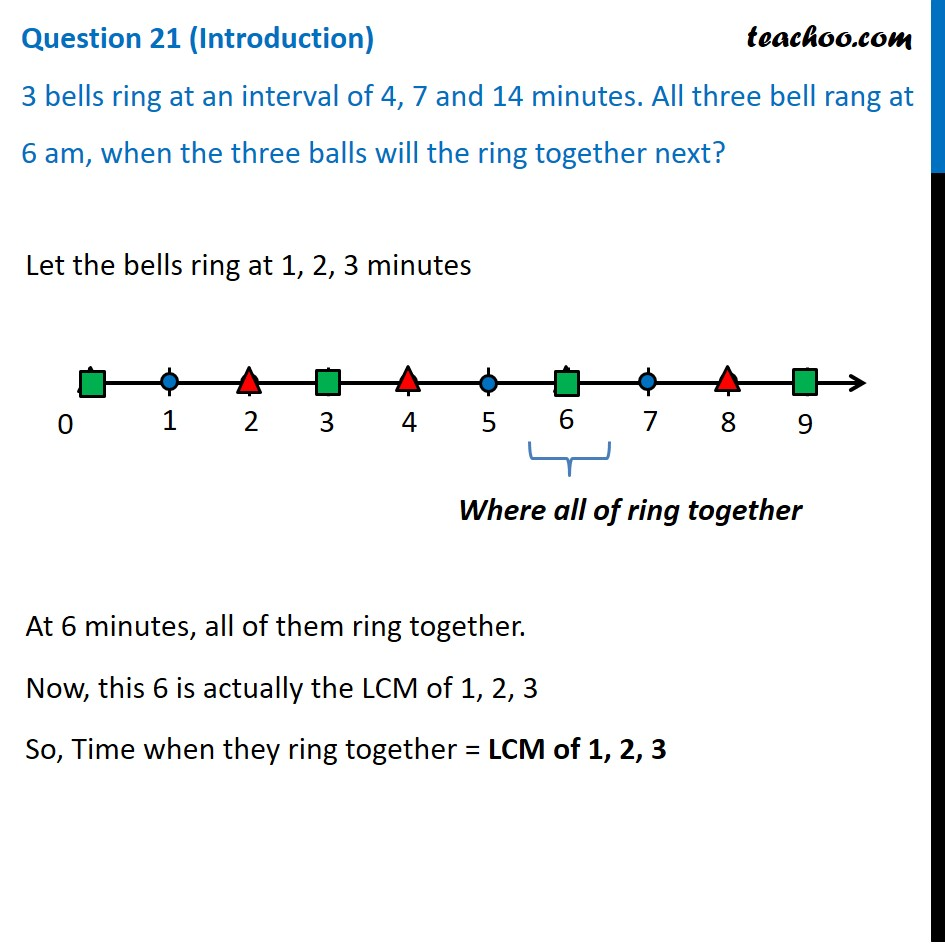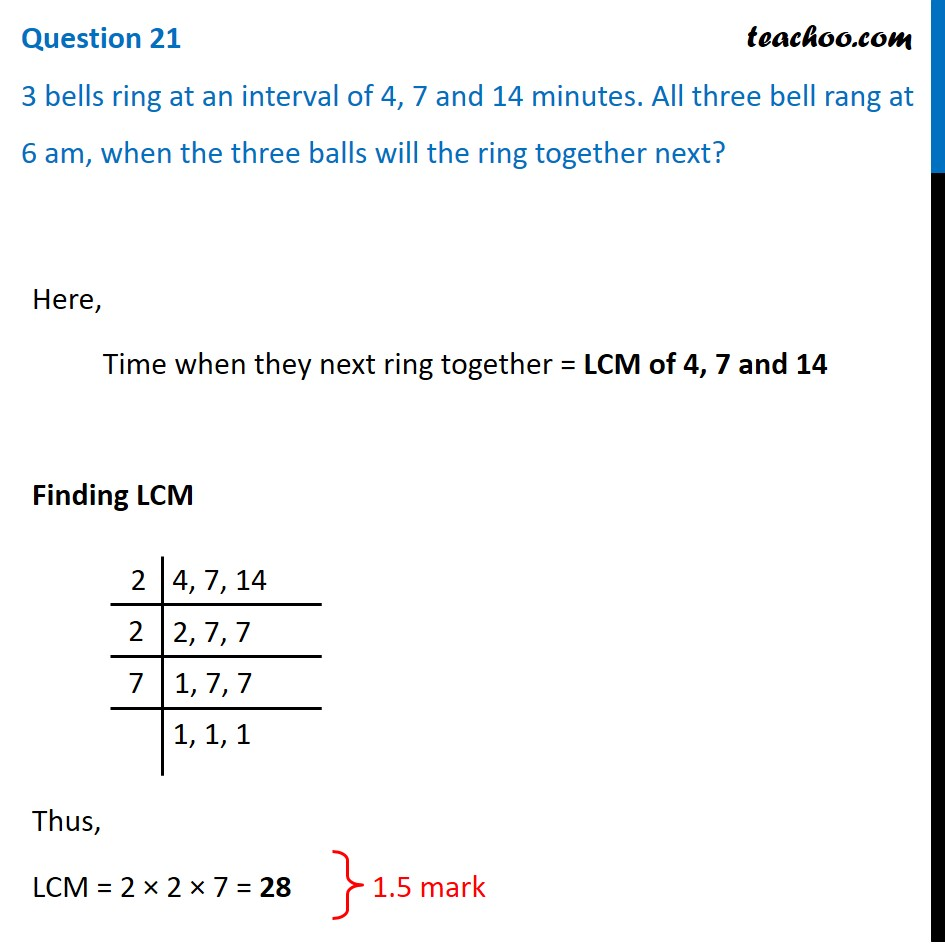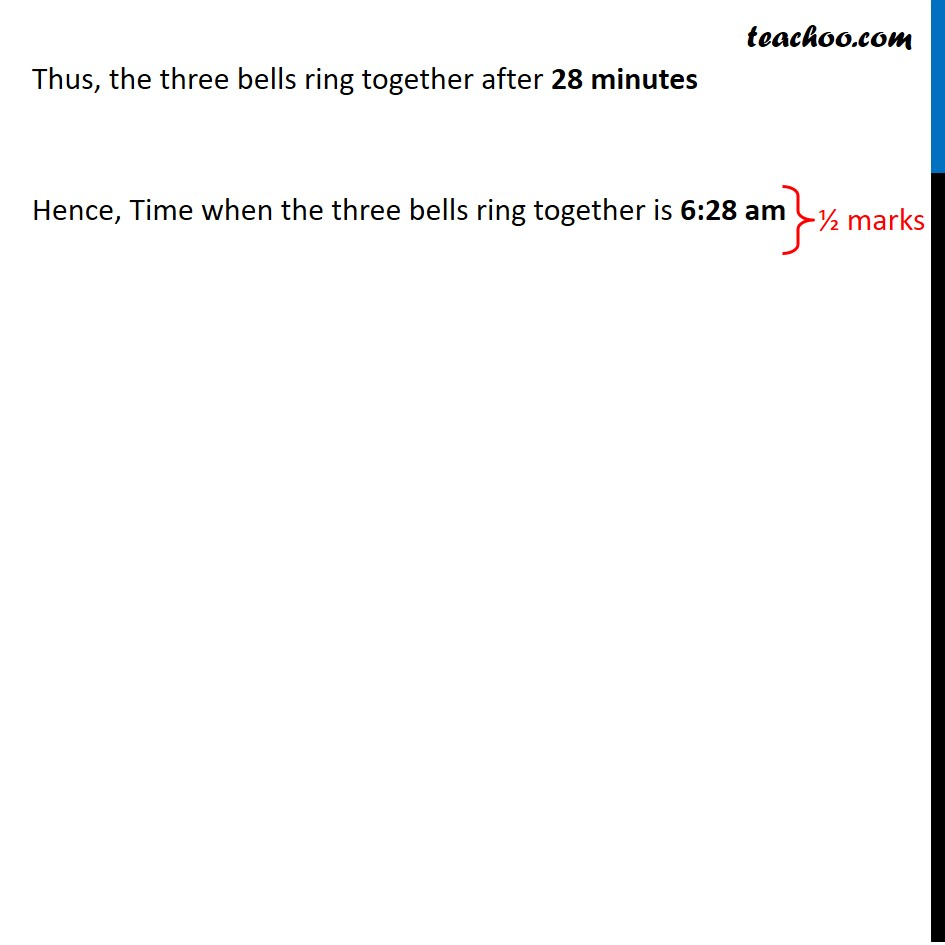## 3 bells ring at an interval of 4, 7 and 14 minutes. All three bell rang at 6 am, when the three balls will the ring together next?1. Class 10
2. Solutions of Sample Papers for Class 10 Boards
3. CBSE Class 10 Sample Paper for 2021 Boards - Maths Standard

Transcript

Question 21 (Introduction) 3 bells ring at an interval of 4, 7 and 14 minutes. All three bell rang at 6 am, when the three balls will the ring together next? Let the bells ring at 1, 2, 3 minutes At 6 minutes, all of them ring together. Now, this 6 is actually the LCM of 1, 2, 3 So, Time when they ring together = LCM of 1, 2, 3 Question 21 3 bells ring at an interval of 4, 7 and 14 minutes. All three bell rang at 6 am, when the three balls will the ring together next? Here, Time when they next ring together = LCM of 4, 7 and 14 Finding LCM Thus, LCM = 2 × 2 × 7 = 28 Thus, the three bells ring together after 28 minutes Hence, Time when the three bells ring together is 6:28 am

CBSE Class 10 Sample Paper for 2021 Boards - Maths Standard

Class 10
Solutions of Sample Papers for Class 10 Boards